# Golf Swing Physics

## 2. The swing

Guest article by Rod White  --  December 2008

## Double-Pendulum Swing

Now we move on the model of the swing itself. Conventionally, physicists model it as a double pendulum: one pendulum tacked onto the end of another.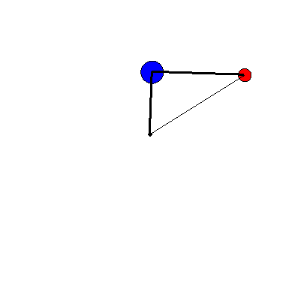In this animation we show a double pendulum composed of a large blue mass representing the arms of the golfer, and a smaller red mass representing the clubhead. There is also a thin string connecting the clubhead to the hub.

Initially the system rotates at a constant speed, and the string stops the clubhead from swinging out. No energy is put into the system for this model; the system is rotating to begin with, and continues to rotate under its own inertia. Since it is held in the same shape by the string, the moment of inertia stays the same so the speed stays the same. An essential feature of the animations is that no external forces or torques are applied to the system, and the connection between the two arms of the pendulum is simply a hinge.

Now watch what happens when the string is removed. As the red mass swings out, the blue mass slows down, and the red mass accelerates. When the system is fully extended (near the point where the head would hit the golf ball) the blue mass comes to a dead stop.

For the physicist, this is the magic moment! The blue mass coming to a dead stop means that all of the kinetic energy that was in the blue mass has been transferred to the red mass (the club head); the swinging process is 100% efficient.

Most importantly, there were no external forces applied to make this happen – the system rearranged itself under its own inertia. If this was a golf swing, the golfer would not have to make any effort to make it happen.

In this example, I chose the value of the red mass so the blue ball would come to a dead stop. In general this does not happen – as we will see with the next few animations.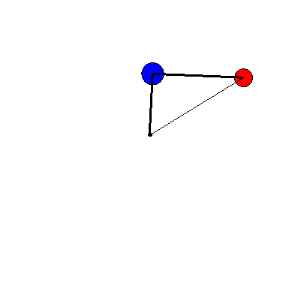The second animation shows the double pendulum again, but with a heavy clubhead mass -- heavier than before and therefore heavier than optimum.

This time, the blue mass slows down more quickly than before, and when the system is fully extended the blue mass actually goes backwards (this tells us that some of the kinetic energy remains in the blue mass).

This animation is similar to the two-ball collision when a small mass collides with the big mass and recoils.The third animation shows the double pendulum again, but with a light clubhead mass. The distribution of mass is closer to a real golf swing than in the previous examples. This time, the blue mass slows down but not as much as before, and when the system is fully extended, the blue mass is still moving forwards. Again some kinetic energy remains in the blue mass.

This animation is similar to the two-ball collision when a large mass collides with the little mass and keeps moving.

Now although the motion of the double pendulum is complicated, we can simplify the problem by considering the initial motion when the string is in place, and later when it is fully extended. If we think about these two snapshots, and ignore the complicated movements as the red ball swings out, we can see a strong similarity between the two-ball collision and the golf swing as it unfolds. Once again we see the Goldilocks principle at work. The red mass representing the club head can be too big, too small or just right. We won’t show you the curve just yet, but it is very similar to the efficiency curve for the 2-ball collision.

The double pendulum is much more complicated than the simple collision, so the condition for a 100% efficient swing is much more complicated that the condition for a 100% efficient collision (which was M1 = M2). We give the equation for the optimum clubhead mass in the math note below.

In addition to the mass of the blue ball representing the arms (M1), the condition depends on the length of the two arms of the pendulum representing the golfer's arm and the shaft of the club (L1 and L2), and the angle between the arm and the shaft (θ, which is the wrist cock angle).

The equation also tells us how to reduce the optimum clubhead mass and increase the efficiency of the swing:

• Lengthen the shaft, and/or
• Increase the wrist-cock angle.
In fact the whole golf stroke: the unfolding of the swing, and the club-ball collision looks a lot like a three-ball collision.

The equations of motion for the system are still complicated, but if we restrict ourselves to thinking only about the energy and momentum at these two points (the point the string is released, and the point of full extension), the system can be understood relatively easily.

Remember that the energy and momentum are the same throughout the swing and through impact. The momentum must be the same; that's physics. The energy is the same because we have not yet considered energy losses in the collision (that is, the COR is 1.00 for our model).

### Math noteHere is the basis of the mathematical model I am using. The diagram shows how I’ve defined the various angles, shaft lengths, etc.

We are only considering the instantaneous motion of the system at the time of two snapshots:
1. The moment just before the string is released. At this moment, everything is rotating; nothing is flying out from the centre of rotation -- though it will the instant after the string is removed.
2. The moment of impact, calculated to correspond to "complete release" -- a straight line from the golfer's center of rotation down the arms and the club to the ball.
By picking these two snapshots, and having the system move under its own inertia, the system is very much simpler to analyze. Mainly, there are no radial terms to consider; only tangential terms involving angular velocities.

Only two things you really need to note here, both of them at the moment the string is released:
• The downswing angle ß, which measures how far the arms move, and
• The wrist cock angle θ, which measures the angle between the arms and the shaft of the club.
These are the simplified equations for the swing. (We still have not included the collision with the ball at this point.) They might still look horrific, but they are much simpler than the full equations, and they can be solved algebraically to give a simple expression for the condition for 100% efficiency.

To read the equations you need to know that terms of the form mass x length2 measure a quantity called the moment of inertia. Moment of inertia measures the resistance to rotational forces (torques) in the same way that mass is a measure of resistance to a translational force. Everybody knows "F=ma". Well the rotational analogue is "torque = moment of inertia x angular acceleration".

The Greek symbols with dots over them are angular velocities.
 Kinetic energies have the form: moment of inertia x (angular velocity)2 Momenta have the form: moment of inertia x angular velocity
The subscripts on the angles, i and f, indicate initial and final velocity.

First, we require the kinetic energy and angular momentum to be the same for the two snapshots of the swing.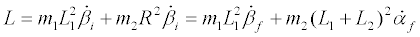Note that the angular velocity of the two masses, beta-dot and alpha-dot, is the same in the first snapshot.

As we did with the equivalent equations for the collision, we can solve these two equations to calculate the final angular velocities for the two masses. This is how the swing efficiency curve was calculated. However, it is more interesting to find out the condition for a 100% efficient swing. Ideally, we want the blue mass to come to a dead stop at impact, and this leads to a further simplification, as given by the second set of equations.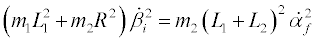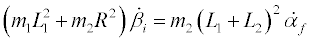This pair of equations is much simpler and has one non-trivial solution:
m1L12 + m2R2  =  m2 (L1 + L2)2

The ‘bottom line’ of this analysis is that the swing is 100% efficient when the moment of inertia of the system in the first snap shot equals the moment of inertia of the club in the second snap shot.

In words, this last equation reads, the moment of inertia of the initial system (with the string in place) equals the moment of inertia of the club when fully extended. This is analogous to the condition that the M1 = M2  in the two-ball collision.

Most importantly, this observation tells us that the energy transfer occurs because of the changing moment of inertia of the club as it swing out.

We can now calculate the optimum clubhead mass for this passive model by substituting the value for R, which is given by the cosine law (from diagram a above):

R2  =  L12 + L22 - 2L1L2 cos θ

Which then gives us the required condition for optimum clubhead mass:

 m2  = m1 2[1 + cos(θ)] L1 L2

## Ice skater analogy of the golf swing

The most important picture to keep in mind is the transfer of energy with unfolding.

Think of this as the reverse of the ice skater effect. The ice skater initially has the arms extended and works to move them as fast as possible. The skater then pulls the arms in close to the body. This causes the body speed up so that the skater spins fast. It is important to note that the hands actually slow down in this process, but we only watch the body. The body is spinning faster, true. But, because the hands' radius of rotation is shorter than before, the velocity of the hands is lower than before.

In the golf swing, the opposite occurs. The golfer initially holds the club close to the body using wrist cock, and works very hard to build up the kinetic energy in the body and arms. The golfer then allows the club to swing away from the body, so the body and arms slow down and the club speeds up. I emphasise the term “allows”, because it can be an entirely passive process; the golfer does not have to make the club swing out – it happens naturally.

In summary...
• The ice skater:
• First builds speed in arms,
• then folds the arms close to the body,
• to speed up the body
• The golfer:
• First builds speed in body,
• then unfolds the club from the body,
• to speed up the club.

## Model golf stroke = pendulum + collision

We are now in  a position to understand the basic principles underlying the golf stroke. There are two parts in the golf stroke: the swing and the collision. This graph is idealised – we discuss the non-ideal bits shortly.Part 2: the collision between the club and ball is exactly as we described for the two-ball collision. The efficiency is represented by the left hand of the two dotted curves, and the maximum efficiency occurs when the two masses are equal (golf ball mass is close to 46 g).

Part 1: the swing is where energy is transferred from the golfer's arms and torso to the clubhead. This is represented by the right hand dotted curve. The right hand dotted curve for the swing efficiency is not exactly the same shape as that for the collision but very close. The exact position of the curve depends on a variety of factors that we haven’t considered in detail yet - arm mass, arm length, shaft length, wrist-cock angle, and coefficient of restitution.

The maximum efficiency in this case for the swing alone requires the club head mass to be about 1 kg (according to the complex equation given in the math notes).  As with the 3-ball collision, the overall efficiency is given by the product of the two separate efficiency curves.

We can now see why the clubhead is so much heavier than the ball. To maximise the efficiency of the total golf stroke, it must be midway between the optimum for the swing (1 kg) and the optimum for the collision (46 g). The right hand dotted curve is not exactly the same shape as the corresponding curve in the three-ball collision – but very similar.

Anything we can do to move the swing curve further left improves the efficiency of the golf stroke. The only two factors included in this model that are within the golfer’s control are:
• The wrist-cock angle (make smaller). That is why many of the longest hitters have a very acute lag angle well into the downswing. (We shall see this in the videos later.)
• Shaft length (make longer). But shaft length is limited by the rules, and in many cases further limited by the individual golfer's ability to control a long club. Long drivers routinely use long shafts, but they too are limited by their competition rules.
The model we have developed so far suggests that the optimum club head mass is about 150 g. In practice, real club-head masses are a little heavier. This is because, unlike our model (which was entirely passive), in a real golf swing, the golfer is constantly working to build up energy and momentum. Work done early in the swing is transferred efficiently, just as our model suggests. Work done late in the swing, when the wrists are un-cocked, is transferred less efficiently. The overall efficiency is therefore less than our model predicts – in effect the average wrist cock angle is greater than we have assumed. There are other factors too:
• Energy lost due to the compression of the ball – as described by the coefficient of restitution – means that the swing is less efficient, and the clubhead mass must be heavier to give the maximum ball speed.
• Our muscles also produce greater torques when they are moving slowly and this favours a slightly heavier mass.
• Finally,the optimum mass changes with the value of m1 that represents the mass of the golfer’s arms, and our value of 1 kg on a 0.66 m pendulum arm may not be representative of the moment of inertia of a typical golfer.